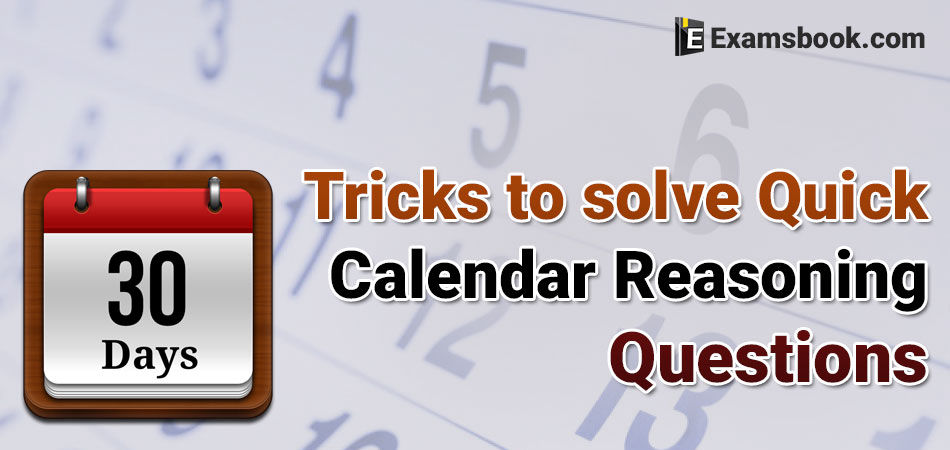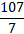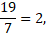• Save

# Calendar Reasoning Questions Tricks for Bank Exams and SSC

Vikram Singh2 years ago 26.5K ViewsDo you face difficulties to solve calendar reasoning questions in competitive exams? Here you can resolve your calendar problems with these calendar reasoning questions tricks for Bank Exams and SSC.

Here you can learn easily how to solve calendar problems quicker with simple tricks. With the help of these tricks, you can improve your score in the competitive exam. You can visit here for more practice of calendar questions with answers and learn how to use calendar problem formulas while solving these questions in the competitive exams.

## Calendar Tricks of Reasoning Questions for Competitive Exams

Q.1. If it was Wednesday on 2 March, then which day would come on 26 March?

(A) Friday

(B) Saturday

(C) Sunday

(D) Tuesday

Solution

Difference between 2 March to 26 March is (26-2) = 24 days. When 24 is divided by 7, we find 3 as a remainder. So, the third day after Wednesday is Saturday. So, it will be Saturday on 26 March.

Q.2. If it is Friday on 10 March 2003, then which day would come on 25 June, 2003 ?

(A) Monday

(B) Tuesday

(C) Sunday

(D) Wednesday

Solution

Difference from 10 March, 2003 to 25 June, 2003 = March+April+May+June = 21+30+31+25=107

Keep in mind ! Only one date from 10 March & 25 June will be accounted, so, for the convenience, we consider 25 June. So, days in March will be (31-10)=21 is written.

Now, we divide the difference obtained by 7, which is := 15 & 2 is the remainder.

So, the day on 25 June will be 2nd next day after Friday, which is Sunday.

Second Method:

Here, we can solve this by writing the odd days only ( the remainder found after dividing by 7) as below :

March + April + May +June

0 + 2 + 3 + 4 = 9

So,= 1, remainder = 2

So, the day after 2 days will be Sunday.

Note: Here we have already divided the days of months by 7. The month of 30 days will give the remainder 2 & similarly, for 31 day’s month, we get remainder 3. In the month o March, we have 21 days as 10 days are not be accounted. So, here we’ll get remainder 0. So, for March, we’ve written 0 here. Similarly, we’ll get remainder 4 for June as we’ve to consider 25 days in June.

The remainders found for each month in normal case are given below :

 January – 3 February – 0,1 March – 3 April – 2 May – 3 June – 2 July – 3 August – 3 September – 2 October – 3 November – 2 December – 3

We can easily & quickly solve the problems if we remember the above table.

Q.3. If it is Wednesday on 17 March, 2005, then which day would come on 12 September, 2005?

(A) Monday

(B) Friday

(C) Sunday

(D) Saturday.

Solution

March+April+May+June+July+Aug+September = 0+2+3+2+3+3+5 = 18.

The remainder will be 4 when 18 is divided by 7.

So, the next 4th day from Wednesday is Sunday.

Note- In the given problem, 17 days of March are not considered, So the remaining days will be 31-17=14. Now, the remainder is 0 when 14 is divided by 7. So, we’ve written 0 for March. Similarly, we’ve to consider only 12 days in September. The remainder will be 5 when 12 is divided by 7, so we’ve written 5 for September.

Q.4. Which day would come on 5 July, 1999 if it was Sunday on 20 November, 1999?

(A) Thursday

(B) Monday

(C) Tuesday

(D) Wednesday

Solution

July+Aug+Sept+Oct+Nov

5+3+2+3+6=19

Then,Remainder = 5.

Here, we have to find the day before given by, so the day before 5 days of Sunday was Tuesday.

We can count (7-5) = 2 next days for our convenience. The 2nd next day of Sunday will be Tuesday.

Note : Here we have written 5 for July as we have to consider (31-5) = 26 & we find remainder 5 when 26 is divided by 7. Similarly, we find 6 as a remainder for November 20 days divided by 7. So, we’ve written 6 for November.

Q.5. If it is Friday on 25 July, 2007; then which was the day on 2 February, 2007?

(A) Tuesday

(B) Sunday

(C) Monday

(D) Wednesday.

Solution

Feb+March+April+May+June+July

5+3+2+3+2+4 = 19

When 19 is divided by 7, we get 2 full cycles (weeks) & 5 as remainder. So 5 days back from Friday OR 2 days after Friday would be Sunday. 1

When there is a difference of so many years between given dates, then

* If there is a difference of 1 year between normal years, then there will be increase or decrease of 1 day.

* If there is a difference of 1 year between leap years, then there will be increase or decrease of 2 days.

Q.6. If it is Friday on 12 April, 2002, then which day would come on 12 April, 2003 ?

(A) Sunday

(B) Saturday

(C) Thursday

(D) None of these.

Solution

Here , 1 normal year is completed. It will be so much time consuming if we write the months during the year.

Here, the difference is of 1 year, so the 1 day after Friday will be Saturday.

Q.7. Pramila was born on 12 January, 1996, Friday. On which day she would celebrate her next birthday?

(A) Monday

(B) Saturday

(C) Sunday

(D) Friday

Solution

1996 is a leap year, so we’ve to count 2 days after the given day for the next year. So, the 2nd next day of Friday is Sunday.

Q.8. 2 days before the yesterday, it was Tuesday, then which would be the day on the day after 2 days from tomorrow?

(A) Wednesday

(B) Tuesday

(C) Monday

(D) Sunday

Solution

2nd Method : Here, difference = before 3 days + after 3 days = 6 days, so it will be Monday after 6 days of Tuesday.

Q.9. Suman remembers that her brother’s birthday comes after 15 February, but before 18 February, whereas her sister remembers that her brother’s birthday comes after 16 January but before 19 February. On which date Suman’s brother’s birthday comes?

(A) 17

(B) 18

(C) 16

(D) 19

Solution

As per Suman – 16, 17

As per Sister – 17, 18

17th is a common date as per both. So her brother’s birthday comes on 17 February.

Q.10. If  a number is larger than 5 but smaller than 9 & larger than 7 but smaller than 11, then find that number.

(A) 5

(B) 6

(C) 7

(D) 8

Solution

As per the 1st condition – 6, 7, 8

As per the 2nd condition – 8,9,10

* is common as per both the conditions, so the number is 8.

I really hope that these calendar reasoning questions tricks are useful for your preparation or competitive exams. If you have any problems related to calendar reasoning questions tricks, you can ask me in the comment section without hesitation.

### Vikram Singh

Providing knowledgable questions of Reasoning and Aptitude for the competitive exams.

## Report Error: Calendar Reasoning Questions Tricks for Bank Exams and SSC